# The Product Rule The Product Rule The product

• Slides: 17The Product Rule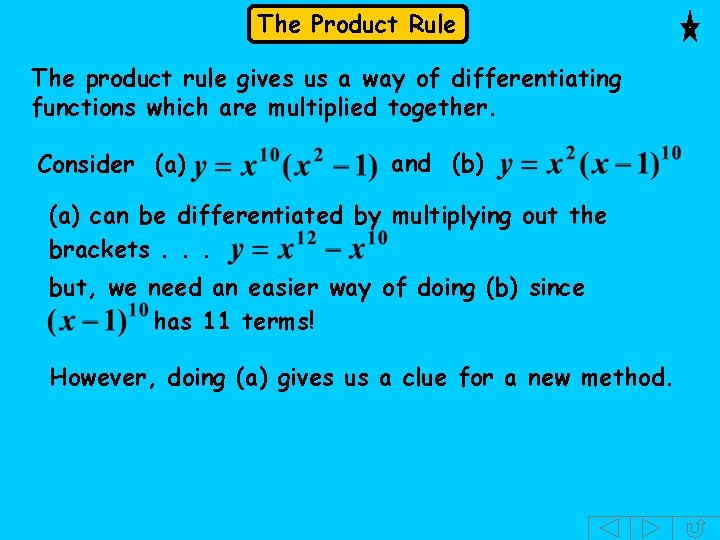The Product Rule The product rule gives us a way of differentiating functions which are multiplied together. Consider (a) and (b) (a) can be differentiated by multiplying out the brackets. . . but, we need an easier way of doing (b) since has 11 terms! However, doing (a) gives us a clue for a new method.The Product Rule (a) Multiplying out: Now suppose we differentiate the 2 functions in without multiplying them out. Let and Multiplying these 2 answers does NOT give BUTThe Product Rule (a) Multiplying out: Now suppose we differentiate the 2 functions in without multiplying them out. Let BUT and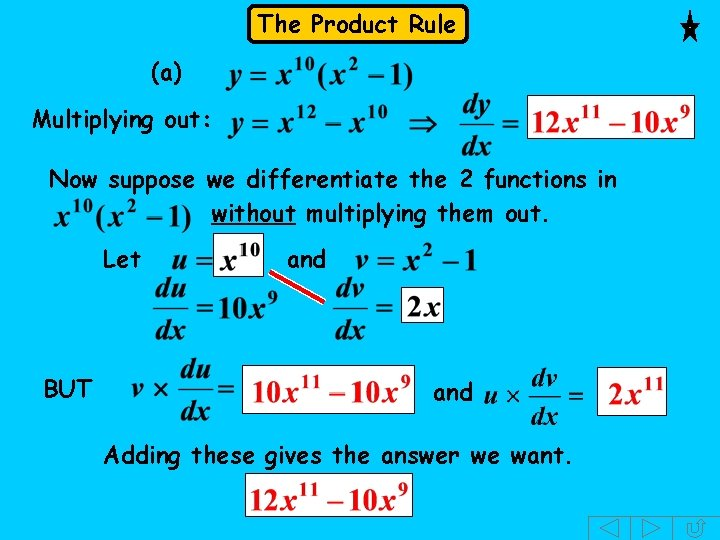The Product Rule (a) Multiplying out: Now suppose we differentiate the 2 functions in without multiplying them out. Let BUT and Adding these gives the answer we want.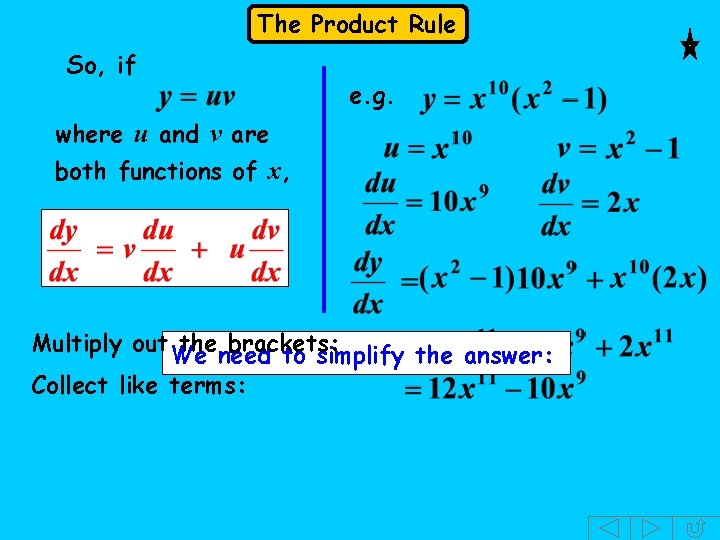The Product Rule So, if e. g. where u and v are both functions of x, Multiply out the brackets: We need to simplify the answer: Collect like terms:SUMMARY The Product Rule To differentiate a product: Ø Check if it is possible to multiply out. If so, do it and differentiate each term. Ø Otherwise use the product rule: where u and v are both functions of x If The product rule says: • multiply the 2 nd factor by the derivative of the 1 st. • Then add the 1 st factor multiplied by the derivative of the 2 nd.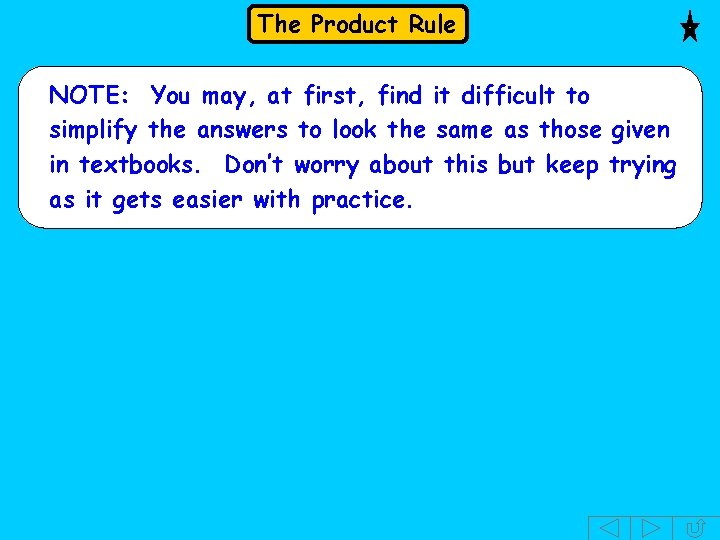The Product Rule NOTE: You may, at first, find it difficult to simplify the answers to look the same as those given in textbooks. Don’t worry about this but keep trying as it gets easier with practice.The Quotient Rule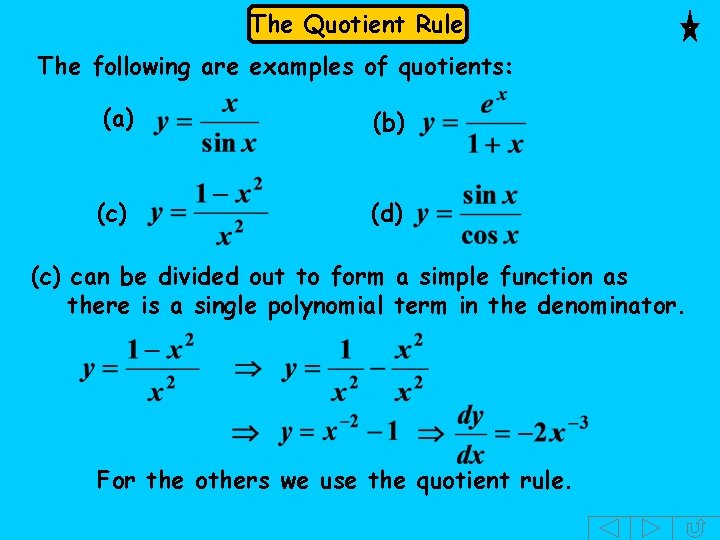The Quotient Rule The following are examples of quotients: (a) (b) (c) (d) (c) can be divided out to form a simple function as there is a single polynomial term in the denominator. For the others we use the quotient rule.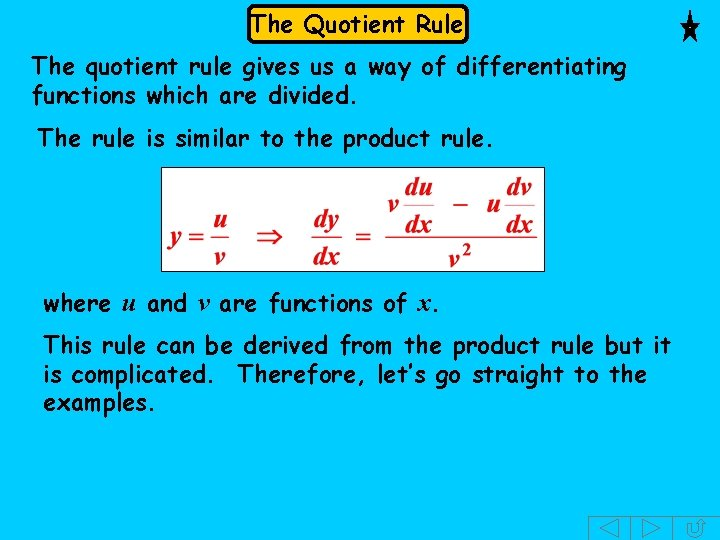The Quotient Rule The quotient rule gives us a way of differentiating functions which are divided. The rule is similar to the product rule. where u and v are functions of x. This rule can be derived from the product rule but it is complicated. Therefore, let’s go straight to the examples.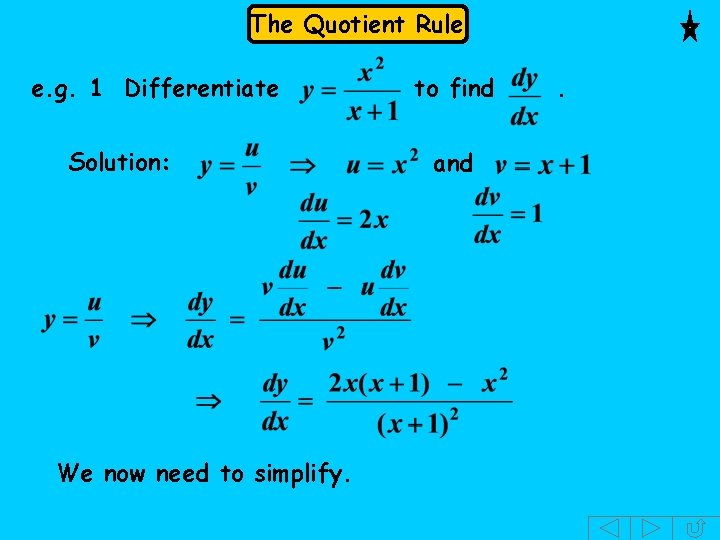The Quotient Rule e. g. 1 Differentiate Solution: We now need to simplify. to find and .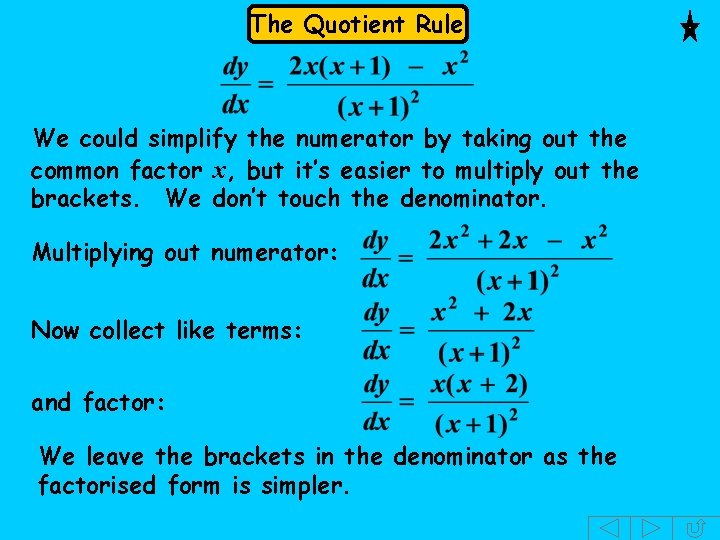The Quotient Rule We could simplify the numerator by taking out the common factor x, but it’s easier to multiply out the brackets. We don’t touch the denominator. Multiplying out numerator: Now collect like terms: and factor: We leave the brackets in the denominator as the factorised form is simpler.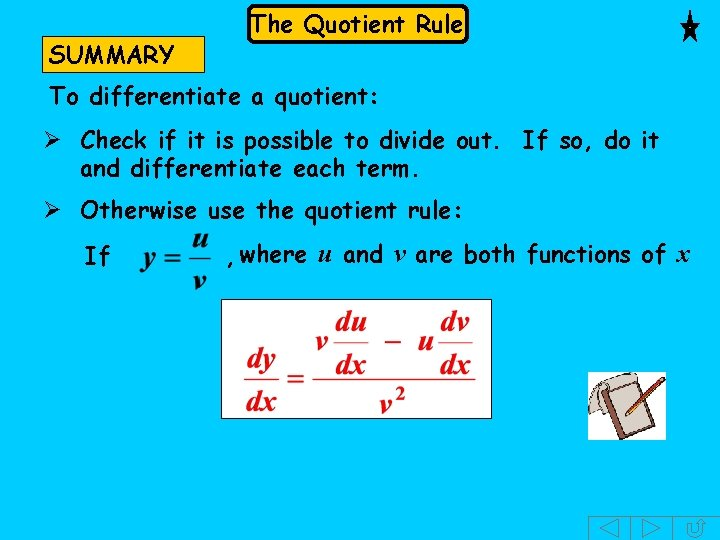SUMMARY The Quotient Rule To differentiate a quotient: Ø Check if it is possible to divide out. If so, do it and differentiate each term. Ø Otherwise use the quotient rule: If , where u and v are both functions of x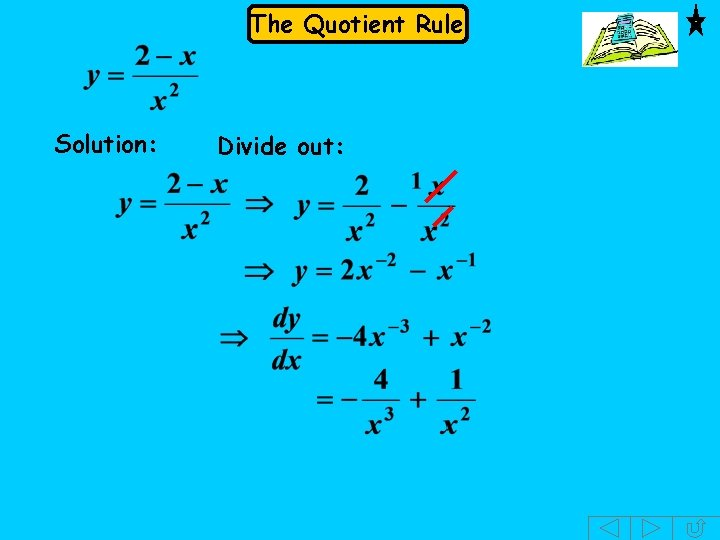The Quotient Rule Solution: Divide out: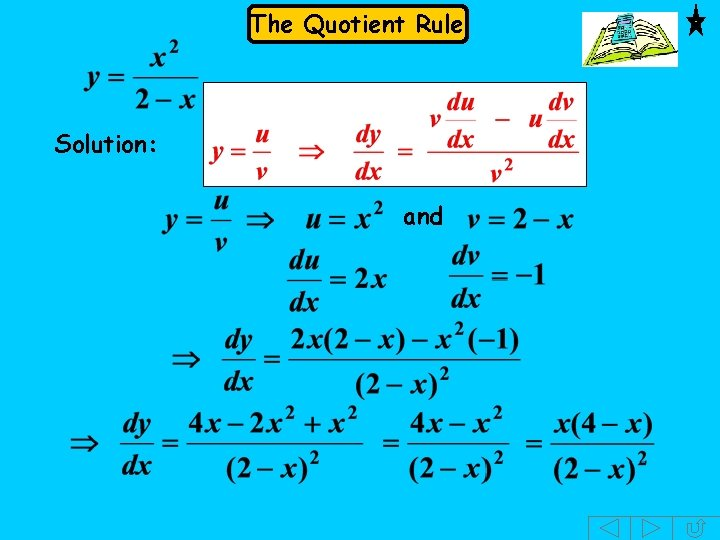The Quotient Rule Solution: and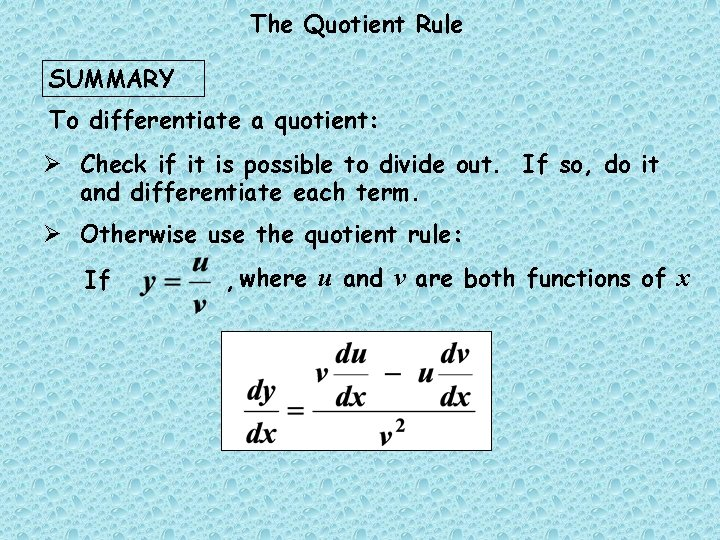The Quotient Rule SUMMARY To differentiate a quotient: Ø Check if it is possible to divide out. If so, do it and differentiate each term. Ø Otherwise use the quotient rule: If , where u and v are both functions of x